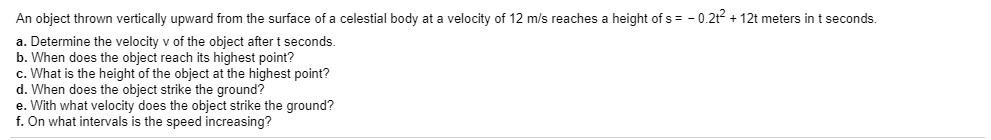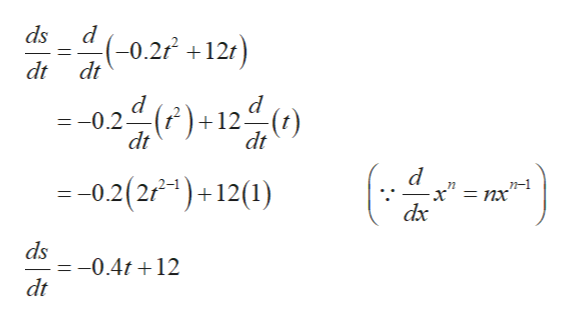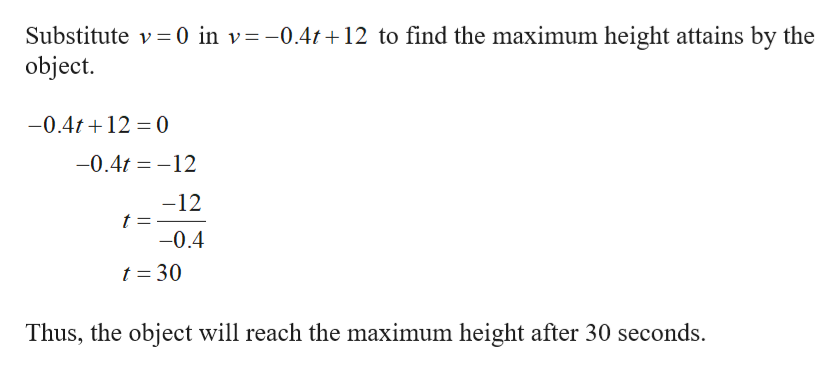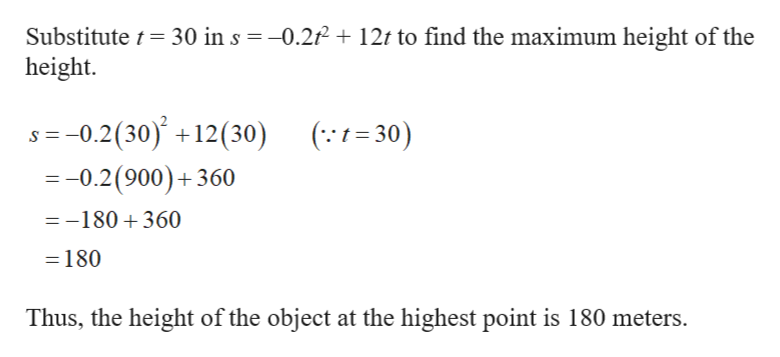# An object thrown vertically upward from the surface of a celestial body at a velocity of 12 m/s reaches a height of s-0.2t12t meters in t seconds.a. Determine the velocity v of the object after t seconds.b. When does the object reach its highest point?c. What is the height of the object at the highest point?d. When does the object strike the ground?e. With what velocity does the object strike the ground?f. On what intervals is the speed increasing?

Question
469 viewshelp_outlineImage TranscriptioncloseAn object thrown vertically upward from the surface of a celestial body at a velocity of 12 m/s reaches a height of s -0.2t12t meters in t seconds. a. Determine the velocity v of the object after t seconds. b. When does the object reach its highest point? c. What is the height of the object at the highest point? d. When does the object strike the ground? e. With what velocity does the object strike the ground? f. On what intervals is the speed increasing? fullscreen
check_circle

star
star
star
star
star
1 Rating
Step 1

The given equation is s = –0.2t2 + 12t and the velocity is v =12 m/s.

a.

Obtain the velocity v of the object after t seconds.

Differentiate s = –0.2t2 + 12t with respect to t as follows.help_outlineImage Transcriptioncloseds (-0.2 +121) dt dt 22()+12 d(1) = - d =-0.2(2)+12(1) n-1 = nx dx ds -0.4t12 dt fullscreen
Step 2

b.

It is known that, if the object attains the maximum then the velocity becomes zero.help_outlineImage TranscriptioncloseSubstitute v 0 in v=-0.4r+12 to find the maximum height attains by the object. -0.4t12 0 -0.4t12 -12 t = -0.4 t = 30 Thus, the object will reach the maximum height after 30 seconds. fullscreen
Step 3

c.

Find the height of the objec...help_outlineImage TranscriptioncloseSubstitute t height. 30 in s -0.2212t to find the maximum height of the = -0.2(30) +12(30) (:1= 30) -0.2(900)+360 =-180 360 180 Thus, the height of the object at the highest point is 180 meters. fullscreen

### Want to see the full answer?

See Solution

#### Want to see this answer and more?

Solutions are written by subject experts who are available 24/7. Questions are typically answered within 1 hour.*

See Solution
*Response times may vary by subject and question.
Tagged in

### Calculus